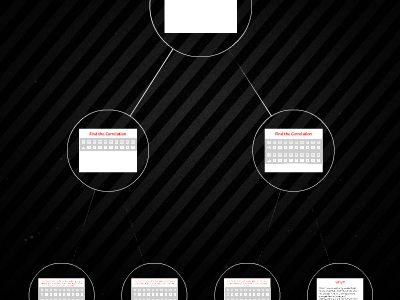Publications: 87 | Followers: 1

## Chapter 3_ Correlation Transformation Investigation

Publish on Category: Birds 0

Chapter3:CorrelationTransformation Investigation
Find the Correlation
R = 0.97

Find the Correlation
R = 0.97

Find the Correlation…The person measuring height was off by 2 inches. Each person is actually 2 inches shorter than reported previously.
R = 0.97

Find the Correlation…The scale was incorrect; each person is actually 5 pounds heavier than previously reported.
R = 0.97

Find the Correlation…The scale was incorrect; each person is actually 5 pounds heavier than previously reported.
R = 0.97

Why?!
Since r is calculated using standardized values (z-scores), the correlation value will not change if the units of measure are changed (feet to inches, etc.)Adding a constant to either x or y or both will not change the correlation because neither the standard deviation nor distance from the mean will be impacted.

0

Embed

Share